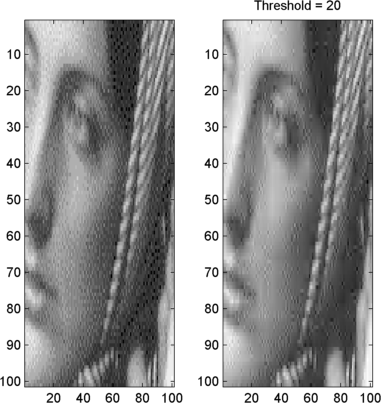## Wavelet Data Compression

The compression features of a given wavelet basis are primarily linked to the relative scarceness of the wavelet domain representation for the signal. The notion behind compression is based on the concept that the regular signal component can be accurately approximated using the following elements: a small number of approximation coefficients (at a suitably chosen level) and some of the detail coefficients.

Like denoising, the compression procedure contains three steps:

1. Decompose

Choose a wavelet, choose a level N. Compute the wavelet decomposition of the signal s at level N.

2. Threshold detail coefficients

For each level from 1 to N, a threshold is selected and hard thresholding is applied to the detail coefficients.

3. Reconstruct

Compute wavelet reconstruction using the original approximation coefficients of level N and the modified detail coefficients of levels from 1 to N.

The difference of the denoising procedure is found in step 2. There are two compression approaches available. The first consists of taking the wavelet expansion of the signal and keeping the largest absolute value coefficients. In this case, you can set a global threshold, a compression performance, or a relative square norm recovery performance.

Thus, only a single parameter needs to be selected. The second approach consists of applying visually determined level-dependent thresholds.

Let us examine two real-life examples of compression using global thresholding, for a given and unoptimized wavelet choice, to produce a nearly complete square norm recovery for a signal (see Signal Compression) and for an image (see Image Compression).

```% Load electrical signal and select a part. load leleccum; indx = 2600:3100; x = leleccum(indx); % Perform wavelet decomposition of the signal. n = 3; w = 'db3'; [c,l] = wavedec(x,n,w); % Compress using a fixed threshold. thr = 35; keepapp = 1; [xd,cxd,lxd,perf0,perfl2] = ... wdencmp('gbl',c,l,w,n,thr,'h',keepapp); ```

Signal CompressionThe result is quite satisfactory, not only because of the norm recovery criterion, but also on a visual perception point of view. The reconstruction uses only 15% of the coefficients.

```% Load original image. load woman; x = X(100:200,100:200); nbc = size(map,1); % Wavelet decomposition of x. n = 5; w = 'sym2'; [c,l] = wavedec2(x,n,w); % Wavelet coefficients thresholding. thr = 20; keepapp = 1; [xd,cxd,lxd,perf0,perfl2] = ... wdencmp('gbl',c,l,w,n,thr,'h',keepapp); ```

Image CompressionIf the wavelet representation is too dense, similar strategies can be used in the wavelet packet framework to obtain a sparser representation. You can then determine the best decomposition with respect to a suitably selected entropy-like criterion, which corresponds to the selected purpose (denoising or compression).

### Compression Scores

When compressing using orthogonal wavelets, the Retained energy in percentage is defined by

When compressing using biorthogonal wavelets, the previous definition is not convenient. We use instead the Energy ratio in percentage defined by

and as a tuning parameter the Norm cfs recovery defined by

The Number of zeros in percentage is defined by

##### SupportGet trial now